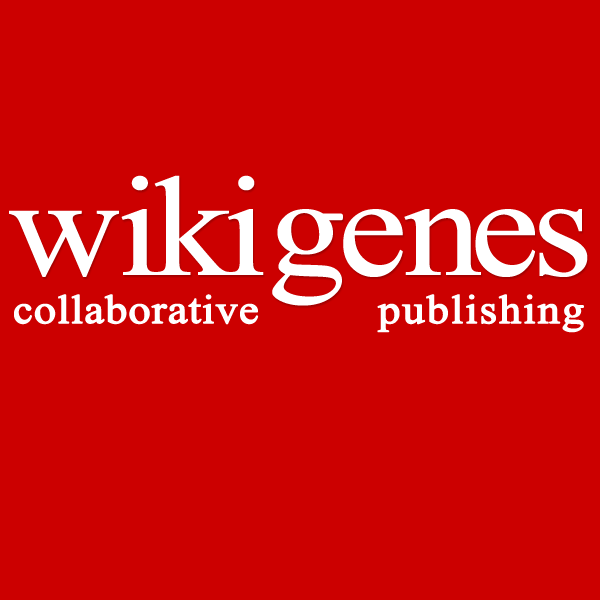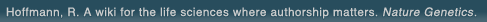# The world's first wiki where authorship really matters (Nature Genetics, 2008). Due credit and reputation for authors. Imagine a global collaborative knowledge base for original thoughts. Search thousands of articles and collaborate with scientists around the globe.

wikigene or wiki gene protein drug chemical gene disease author authorship tracking collaborative publishing evolutionary knowledge reputation system wiki2.0 global collaboration genes proteins drugs chemicals diseases compound
Hoffmann, R. A wiki for the life sciences where authorship matters. Nature Genetics (2008)# Structure of the adipate complex [Na2(C6H9O4)2(C6H10O4)].2H2O from neutron diffraction at 220 and 295 K.

The crystal structure of sodium hydrogen adipate-adipic acid (2/1) dihydrate, [Na2(C6H9O4)2(C6H10O4)].2H2O, Mr = 518.4, has been determined from neutron diffraction data collected at 220 and 295 K. Crystals are monoclinic, space group C2/m, with Z = 2. At 295 K, a = 9.378 (2), b = 13.379 (5), c = 10.247 (3) A, beta = 95.93 (3) degrees, V = 1278.8 (7) A3, Dn = 1.346, Dm = 1.348 (1) g cm-3 (in dibromomethane/bromobutane), lambda = 1.1588 (2) A, mu = 2.186 cm-1, F(000) = 176.4 fm, R(F2) = 0.108 for all 1995 nonequivalent reflections with sin theta/lambda less than 0.71 A-1. The crystal structure is similar at 220 K except for reduced nuclear mean-square displacements. Hydrogen adipate subunits (called A) are linked end-to-end in infinite chains by very short O...O (2.44 A) hydrogen bonds where the H nuclei are on centers of symmetry within the experimental error. The Na cation is octahedrally coordinated by O atoms from molecules A and also by non-ionized adipic acid molecules (called B). The B molecules have large mean-square nuclear displacements which are described in terms of anharmonicity and disorder.

## References

1. Structure of the adipate complex [Na2(C6H9O4)2(C6H10O4)].2H2O from neutron diffraction at 220 and 295 K. Gao, Q., Clancy, L., Weber, H.P., Craven, B.M., McMullan, R.K. Acta Crystallogr., B (1991) [Pubmed]